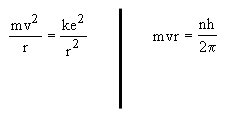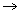# Physics HomeworkPanda

Solve the equation on the far right for v. Then substitute this expression for "v" into the first set of equations. Now solve for "r," the radius of the discrete orbit.Calculate the orbital radius of the hydrogen atom for the first principal quantum number. Use the general expression given in the test to calculate this value. (Hint: Quantum numbers are not significant digits and should not be counted as such in determining your final answer. Thus, this answer should have 2 significant digits.)

[removed] A

Calculate the speed of an electron in the innermost orbit of a hydrogen atom.

[removed] x 10[removed] m/sec

Use the Energy Levels for Hydrogen chart in the text to calculate the wavelength corresponding to the following electron transition. (Due to significant figure rules, energy answers should be written to the nearest tenth and wavelengths should have 2 significant figures.)

 Transition Energy in ev's Emitted wavelengths in m 31 [removed]a0 [removed]a1 x 10[removed]a2

Calculate the orbital radius of the hydrogen atom for the second principal quantum number.

[removed] A

Calculate the orbital radius of the hydrogen atom for the fifth principal quantum number.

[removed] A

Assume that the radius of the hydrogen nucleus is 1.4 · 10-15 meters. How much larger than the nucleus is the entire hydrogen atom? (Calculate the atomic radius for n = 1. Round answer to nearest tenth.)

[removed] x 10[removed] times larger than the nucleus.

A pure sample of atomic gas has atoms with six principal quantum numbers that can yield several emission lines. What is the number of possible electron shell transitions for which energy is radiated? (Hint: if an electron has jumped form n = 1 to n = 6, it can have these transitions: n=6 to n=5, n=6 to n=4, n=6 to n=3, n=6 to n=2, n=6 to n=1. That's 5 of them – can you find the rest? Remember, the electron didn't have to jump all the way to the n=6 level to start out with.)

[removed]

Use the Energy Levels for Hydrogen chart in the text to calculate the wavelength corresponding to the following electron transition. (Due to significant figure rules, energy answers should be written to the nearest tenth and wavelengths should have 2 significant figures.)

 Transition Energy in ev's Emitted wavelengths in m 21 [removed] [removed] x 10[removed]

• 8 years ago
• 20
•